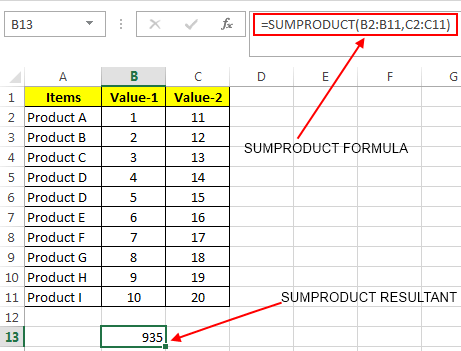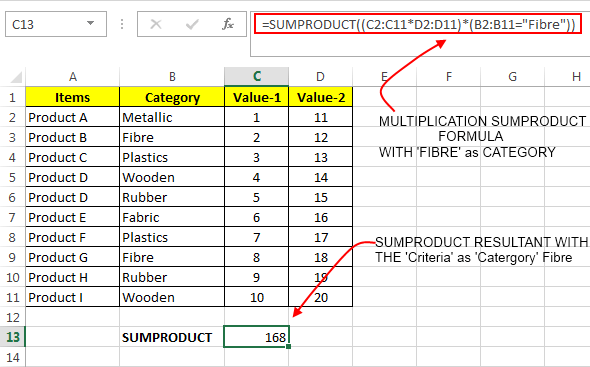# Excel SUMPRODUCT Function – How To Use

On hearing the name of Excel SUMPRODUCT Function for the first time, it looks like some useless formula that can do some predefined sum and product operations. But actually it’s far from that definition. In fact it is a very useful function if you need to analyse the data of two or multiple arrays in excel.

### How Excel Defines SUMPRODUCT Function:

Microsoft excel defines as SUMPRODUCT Formula as: ‘Returns the sum of the products of corresponding ranges or arrays’. But this function is capable of much more than its definition.

### Syntax of Excel SUMPRODUCT Function:

The basic syntax of this formula is quite simple as shown below:

`SUMPRODUCT(Array1,Array2,Array3, ...ArrayN)`

Here Array1, Array2 and Array3 are the three cell ranges or arrays. All the arrays must have same number of elements. The minimum number of arrays that can be used in the SUMPRODUCT function is 2 and the maximum number is 30.

NOTE: If any array contains non numeric value then it is treated as 0.

### Excel SUMPRODUCT Formula Examples:

If you have a table as shown in the below image:Now if you want to apply SUMPRODUCT formula on these values then your formula should be:

`=SUMPRODUCT(B2:B11,C2:C11)`

This will give you an output = 935. So, internally SUMPRODUCT has multiplied the each element of both the arrays and then added it to one another.

Example 1.: `(1x11)+(2x12)+(3x13)+(4x14)+(5x15)+(6x16)+(7x17)+(8x18)+(9x19)+(10x20) = 935`

### SUMPRODUCT FUNCTION by Changing Arithmetic Operators:

This is another good thing about the SUMPRODUCT formula. SUMPRODUCT can also be used with user defined mathematical operators instead of the default multiplication operation. An example will make this clear:

Writing a MULTIPLICATION SUMPRODCUT function as: `=SUMPRODUCT(B2:B11*C2:C11)` “Replaced comma with multiplication sign”

This makes sense as it tells excel to use the multiplication operator between the corresponding array elements and then add them up (same as Example 1). So it will also yield 935 in the case of above example.

This is the same thing that you can achieve with the formula: `=SUMPRODUCT(B2:B11,C2:C11)`

But, using other mathematical operators in the SUMPRODCUT Function can help you to customise the operation between the array elements.

So, a DIVISION SUMPRODUCT can be used as:`=SUMPRODUCT(B2:B11/C2:C11)`

In the context of above example it will we implemented as: `(1÷11)+(2÷12)+(3÷13)+(4÷14)+(5÷15)+(6÷16)+(7÷17)+(8÷18)+(9÷19)+(10÷20)`

And gives and output = 3.312285968

An ADDITION SUMPRODUCT can be used as: `=SUMPRODUCT(B2:B11+C2:C11)`

And Microsoft Excel will implement it as:

`(1+11)+(2+12)+(3+13)+(4+14)+(5+15)+(6+16)+(7+17)+(8+18)+(9+19)+(10+20)`

And the output will be: 210

Similarly a SUBTRACTION SUMPRODUCT can be used as: `=SUMPRODUCT(B2:B11-C2:C11)`

This will be implemented internally as:

`(1-11)+(2-12)+(3-13)+(4-14)+(5-15)+(6-16)+(7-17)+(8-18)+(9-19)+(10-20)`

And will result into a value = -100

### Using Criteria in SUMPRODUCT Calculation:

Now comes the best part of using the Excel SUMPRODUCT Function. SUMPRODUCT formula can also be used in performing selective data operations based on a particular criteria.For instance: You have a data set as shown in the above image. And you want to perform a MULTIPLICATION SUMPRODUCT of two array ranges but only for the category named ‘Fibre’.

Then the formula should be as: `=SUMPRODUCT((C2:C11*D2:D11)*(B2:B11="Fibre"))`

Excel will search the category ‘fibre’ in the defined range. And will only do the SUMPRODUCT for the values of ‘fibre’ category like: `(2x12)+(8x18)` which gives the result 168.

So, this was all about using SUMPRODUCT Function in Excel.About Ankit Kaul

Ankit is the founder of Excel Trick. He is tech Geek who loves to sit in front of his square headed girlfriend (his PC) all day long. :D. Ankit has a strong passion for learning Microsoft Excel. His only aim is to turn you guys into 'Excel Geeks'.